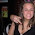## Wednesday, April 21, 2010

### Theo Jansen Mechanism Matlab Code

As promised, here is some of the Matlab code for the Theo Jansen Mechanism we posted a video of a little while back. We begin every Matlab program with the clear all command. Next are the lengths for all of the linkages.

clear all

L1=286;

L2=120;

L3=400;

L4=275;

L5=400;

L6=290;

L7=275;

L8=275;

L9=400;

L10=290;

L11=400;

L12=275;

L13=170;

%To get the video output:

a=1;

mov=avifile('TheoJansen.avi','COMPRESSION','None');

for theta=0:pi/20:8*pi

%Relating all values to theta2 of 4-bar mechanism O2ABO1

theta2=pi-theta;

ssquared=(L2)^2+(L1)^2-(2*L1*L2*cos(theta2));

s=sqrt(ssquared);

beta=asin((L2*sin(theta2))/s);

psi=acos(((L3^2)+(s^2)-(L4^2))/(2*L3*s));

lambda=acos((L4^2+s^2-L3^2)/(2*L4*s));

theta3=pi-psi+beta;

theta4=pi+beta+lambda;

theta5=lambda+beta;

%Finding the points relative to the motion of theta2

O1=[0,0];

O2=[-L1,0];

A=[L2*cos(theta) L2*sin(theta)];

B=[A(1)-L3*cos(psi-beta) A(2)+L3*sin(psi-beta)];

%Plotting the 4-bar mechanism

plot ([O1(1),A(1)],[O1(2),A(2)],'r','linewidth',3)

hold on

plot ([O2(1),O1(1)],[O2(2),O1(2)],'black','linewidth',3)

plot ([A(1),B(1)],[A(2),B(2)],'g','linewidth',3)

plot ([O2(1),B(1)],[O2(2),B(2)],'r','linewidth',3)

%Bottom triangle DEF

F=[E(1)-L12*cos(lambda+beta) E(2)-L12*sin(lambda+beta)];

plot([D(1),F(1)],[D(2),F(2)],'linewidth',3)

plot([E(1),F(1)],[E(2),F(2)],'linewidth',3)

G=[F(1)-L13*cos(lambda+beta) F(2)-L13*sin(lambda+beta)];

%Trajectory of point G

plot([F(1),G(1)],[F(2),G(2)],'linewidth',3)

Gx(a)=G(1);

Gy(a)=G(2);

plot(Gx,Gy)

axis([-800 400 -800 400])

hold off

a=a+1;

pause(.1)

M=getframe;

end

mov=close(mov);

I left out a portion in the middle so you'll just have to figure that out by yourself!

1.I am currently working on this exact project for a class and I am running into a few problems. Would it be possible for you to send me the entire .m file so I could see where I am going wrong? I assure you your work would be used only as a guide and would not be copied.

Thanks

2.I'll have to see if I still have the whole file.

3.This comment has been removed by the author.

4.I am working on this project and having problems as well. And if you would be able to send me the whole .m to be used as a guide also that would be extremely helpful.

Thanks

5.I am working on this same project and I'm probably having the same problems. Defining the angles relative to the input angle and then using that. It would be great though if you could send me the .m file as well.

Thanks

6.I think I've found the complete code. I'll be uploading the file very soon!

7.8.Can u send me ur Matlab code, i can't download it. thanks

9.10.my email is chealmin140@gmail.com
thank you

11.Hello please email my the full math lab code
I am pabllo2@yahoo.fr

12.This is awesome, thank you so much. Could you share how you numbered the links? Or if you have a diagram showing L1 L2 etc I would really appreciate it. Thanks again, this helped me a lot.

13.Can you please send the m.file ? tlg.ercan@hotmail.com

14.Can you please send the m.file ? tlg.ercan@hotmail.com

I'd love to hear from you!